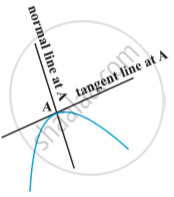# Tangents and Normals

#### notes

The equation of a straight line passing through a given point (x_0, y_0) having finite slope m is given by
y - y_0 = m (x - x_0)
Note that the slope of the tangent to the curve y = f(x) at the point (x_0, y_0) is given by (dy)/(dx)]_(x_0,y_0) (=f'(x_0)).
So the equation of the tangent at (x_0,y_0) to the curve y = f(x) is given by y-y_0 = f'(x_0)(x - x_0)Fig.Also, since the normal is perpendicular to the tangent, the slope of the normal to the curve y = f(x) at  (x_0, y_0) is (-1)/(f'(x_0)) , if  f'(x_0) ≠ 0 . Therefore, the equation of the normal to the curve y = f(x)  at (x_0, y_0)  is given by

y – y_0 = (-1)/(f'(x_0))(x - x_0)

i.e. (y-y_0) f'(x_0) + (x-x_0) = 0

Particular cases:

(i) If slope of the tangent line is zero, then tan θ = 0 and so θ = 0 which means the tangent line is parallel to the x-axis. In this case, the equation of the tangent at the point (x_0, y_0) is given by y = y_0.

(ii) If theta →pi / 2 , then tan θ → ∞, which means the tangent line is perpendicular to the x-axis, i.e., parallel to the y-axis. In this case, the equation of the tangent at (x_0, y_0)  is given by x = x_0

If you would like to contribute notes or other learning material, please submit them using the button below.

#### Video Tutorials

We have provided more than 1 series of video tutorials for some topics to help you get a better understanding of the topic.

Series 1

Series 2

Series 3

### Shaalaa.com

Tangents and Normals part 1 [00:27:13]
S
0%